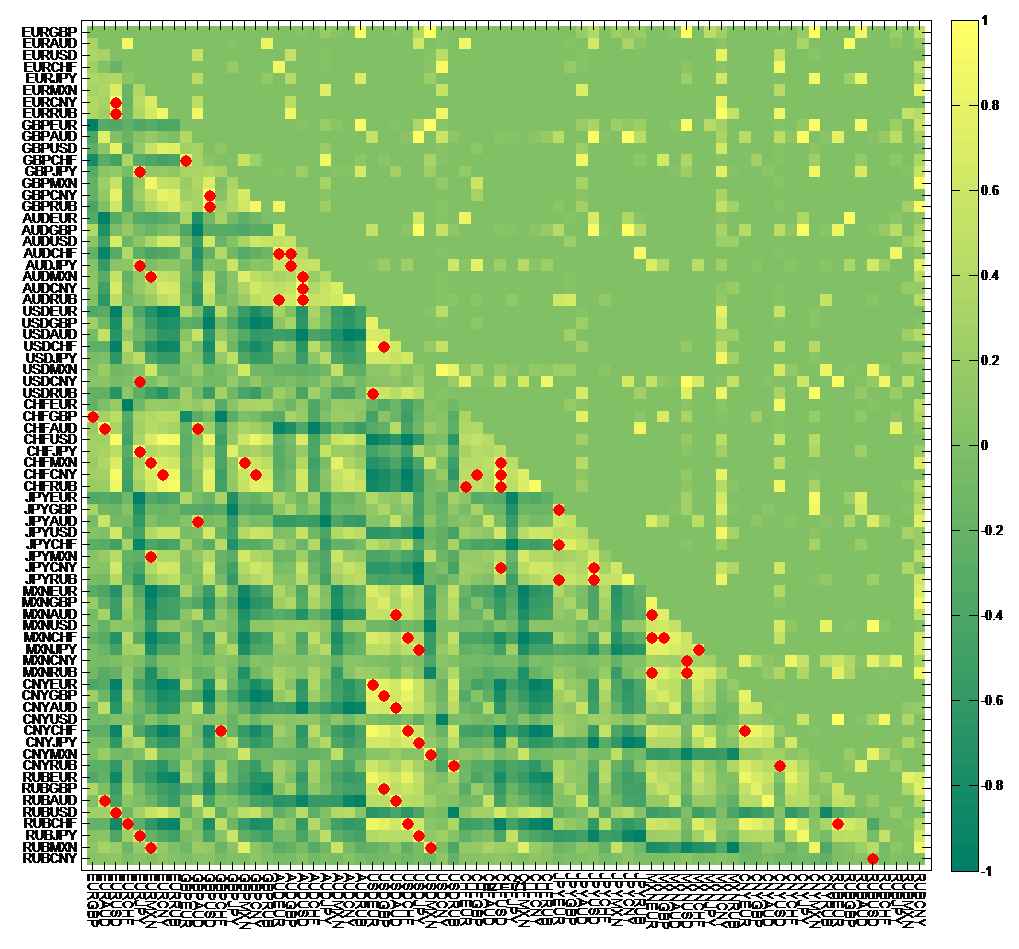# Annex: Derivation of correlation-based minimum spanning trees

Suppose there are n foreign exchange rates, and let$r_{i}$$and r_{j}$ denote vectors of the logarithmic returns of foreign exchange rates$i \in \{1,2,...,n\}$ and$j \in \{1,2,...,n\}$ respectively. The$n \times n$ correlation coefficient matrix as in the lower triangular part of Chart A1 is then derived as follows (1999, Mantegna).$\rho_{ij}=\frac{\langle r_{i}r_{j} \rangle - \langle r_{i} \rangle \langle r_{j} \rangle }{\sqrt{( \langle r_{i}^2 \rangle - \langle r_{i} \rangle ^2)( \langle r_{j}^2 \rangle - \langle r_{j} \rangle ^2)}}$

Chart A1: Correlation coefficient (lower triangular part), adjacency (red dots), and p-value (upper triangular part) matrix of logarithmic returns of daily bilateral exchange rates from 3 January 2005 to 29 December 2006Sources: Bank calculations, BIS, and Bloomberg

As noted above, we can think of the foreign exchange market as a weighted graph where a finite set of vertices denotes foreign exchange rates while a finite set of edges connecting vertices represents correlation coefficients between the foreign exchange rates. In a weighted graph, the weight associated with each edge must be a positive real number, but correlation coefficients can be negative. We therefore transform the correlations into positive real number weights by the map,$d_{ij}:\rho_{ij} \in [-1,1] \mapsto \sqrt{2(1- \rho_{ij})} \in [0,2]$.

Since the transformation,$d_{ij}$, is bijective and monotonically decreasing, a spanning tree,$\bf{T}$, that minimises$\sum\nolimits_{d_{ij} \in \bf{T}} d_{ij}$ necessarily maximises$\sum\nolimits_{\rho_{ij} \in \bf{T}} \rho_{ij}$. Thus an MST for the weights,$d_{ij}$, is a network of the most highly correlated foreign exchange rates by price return.

The number of spanning trees for a complete graph with$n$ vertices is$n^{n-2}$. Thus, it would be computationally inefficient to consider all of the possible spanning trees to derive the MST. Instead, there are two widely used algorithms for deriving the MST, i.e., Prim’s and Kruscal’s. This blog uses the former to derive the MST. The MST has n-1 edges as shown in the adjacency matrix in Chart A1 where the red dots indicate that the corresponding foreign exchange rate pairs are connected in the MST.

This blog plots MSTs graphically using a force-directed graph-drawing algorithm called Kawai-Kamada. And in plotting a series of MSTs for the dynamic MST, the$(x,y)$ -coordinate layout of the previous network is used to calculate that of the new network such that changes in the layout of the dynamic MST is minimised – the author would like to thank Michael Woodford for coding this algorithm, and Will Dison and Tahir Mahmood for reviewing this annex.